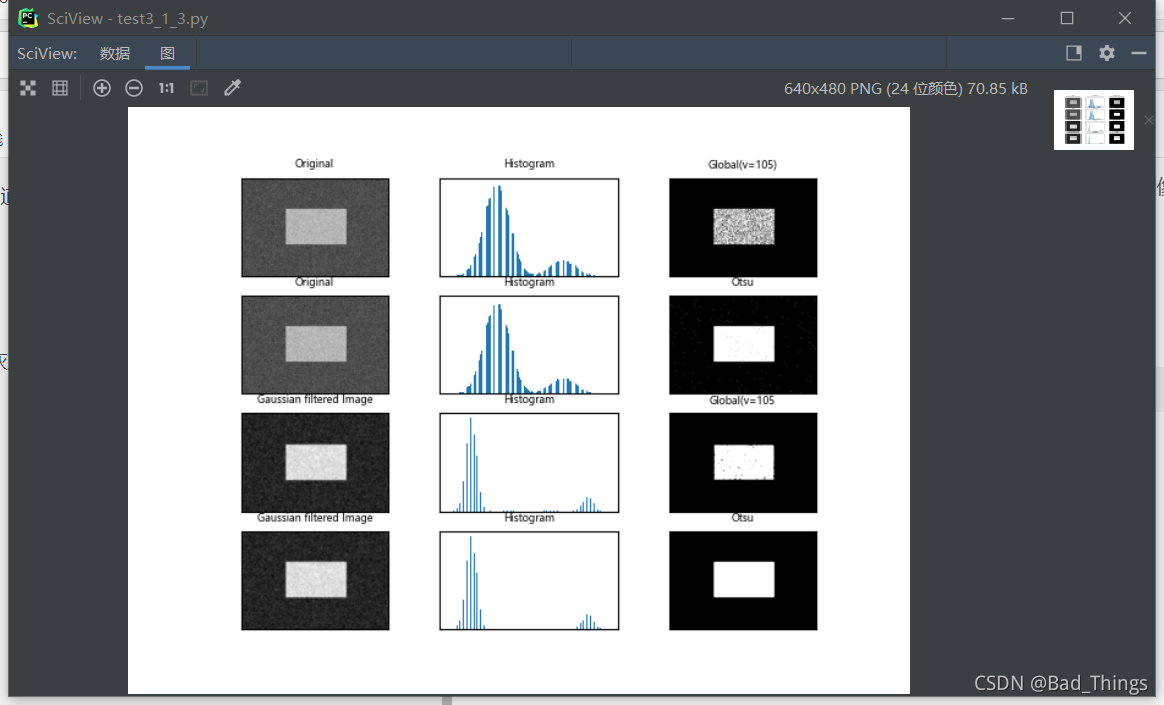# How to Solve Opencv Error: CPP: 1557 error

Opencv Error:
ret2, th2 = cv.threshold(img, 0, 255, cv.THRESH_BINARY + cv.THRESH_OTSU)
cv2.error: OpenCV(4.5.3) C:\Users\runneradmin\AppData\Local\Temp\pip-req-build-c2l3r8zm\opencv\modules\imgproc\src\thresh.cpp:1557: error: (-2:Unspecified error) in function ‘double __cdecl cv::threshold(const class cv::_InputArray &,const class cv::_OutputArray &,double,double,int)’

THRESH_OTSU mode:
‘src_type == CV_8UC1 || src_type == CV_16UC1’
where
‘src_type’ is 16 (CV_8UC3)

Original Codes:

``````img = cv.imread('noisy.jpg')

# Fixed threshold method
ret1, th1 = cv.threshold(img, 105, 255, cv.THRESH_BINARY)
# Otsu threshold method
ret2, th2 = cv.threshold(img, 0, 255, cv.THRESH_BINARY + cv.THRESH_OTSU)
# Perform Gaussian filtering first, and then use Otsu threshold method
blur = cv.GaussianBlur(img, (5, 5), 0)
ret3, th3 = cv.threshold(blur, 105, 255, cv.THRESH_BINARY)
ret4, th4 = cv.threshold(blur, 0, 255, cv.THRESH_BINARY + cv.THRESH_OTSU)
``````

Problem analysis

The CV2.Threshold() function inputs graphics in the form of single channel, while the image in the above code is input in the form of color three channels, resulting in an error.

Solution:

Convert the color image into a single channel gray image for input.

``````img = cv.imread('noisy.jpg',0)
``````

Rerun the program

Run successfully